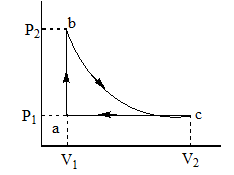Heat given
Question

# Carbon monoxide is carried around a closed cycle abc, in which bc is an isothermal process, as shown in the figure.  The gas absorbs 7000 J of heat, as its temperature is increased from 300K to 1000K in going from a to b.  The quantity of heat rejected by the gas during the process ca isModerate
Solution

## From a to b heat absorbed =  ${\mathrm{nc}}_{\mathrm{v}}\mathrm{dt}$$\frac{\mathrm{n}5\mathrm{R}}{2}\left(1000-300\right)=7000$$\frac{\mathrm{n}5\mathrm{R}}{2}×\left(700\right)=7000$nR = 4In the process c to a at constant pressure temperature decreases from 1000 to 300 K.Heat rejected ${\mathrm{nc}}_{\mathrm{p}}\mathrm{dt}$$\mathrm{n}\frac{7\mathrm{R}}{2}\left(700\right)$$\frac{\mathrm{nR}}{2}×7×700$$\frac{4}{2}×4900=9800\mathrm{J}$

Get Instant Solutions